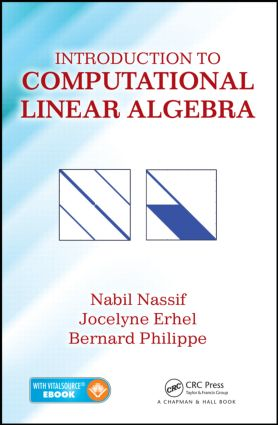# Introduction to Computational Linear Algebra

## 1st Edition

Chapman and Hall/CRC

259 pages | 9 B/W Illus.

##### Purchasing Options:\$ = USD
Hardback: 9781482258691
pub: 2015-06-26
\$94.95
x
eBook (VitalSource) : 9780429190933
pub: 2015-06-24
from \$47.48

FREE Standard Shipping!

### Description

Teach Your Students Both the Mathematics of Numerical Methods and the Art of Computer Programming

Introduction to Computational Linear Algebra presents classroom-tested material on computational linear algebra and its application to numerical solutions of partial and ordinary differential equations. The book is designed for senior undergraduate students in mathematics and engineering as well as first-year graduate students in engineering and computational science.

The text first introduces BLAS operations of types 1, 2, and 3 adapted to a scientific computer environment, specifically MATLAB®. It next covers the basic mathematical tools needed in numerical linear algebra and discusses classical material on Gauss decompositions as well as LU and Cholesky’s factorizations of matrices. The text then shows how to solve linear least squares problems, provides a detailed numerical treatment of the algebraic eigenvalue problem, and discusses (indirect) iterative methods to solve a system of linear equations. The final chapter illustrates how to solve discretized sparse systems of linear equations. Each chapter ends with exercises and computer projects.

Basic Linear Algebra Subprograms: BLAS

An Introductory Example

Matrix Notations

IEEE Floating Point Systems and Computer Arithmetic

Vector-Vector Operations: Level-1 BLAS

Matrix-Vector Operations: Level-2 BLAS

Matrix-Matrix Operations: Level-3 BLAS

Sparse Matrices: Storage and Associated Operations

Basic Concepts for Matrix Computations

Vector Norms

Complements on Square Matrices

Rectangular Matrices: Ranks and Singular Values

Matrix Norms

Gauss Elimination and LU Decompositions of Matrices

Special Matrices for LU Decomposition

Gauss Transforms

Naive LU Decomposition for a Square Matrix with Principal Minor Property (pmp)

Gauss Reduction with Partial Pivoting: PLU Decompositions

MATLAB Commands Related to the LU Decomposition

Condition Number of a Square Matrix

Orthogonal Factorizations and Linear Least Squares Problems

Formulation of Least Squares Problems: Regression Analysis

Existence of Solutions Using Quadratic Forms

Existence of Solutions through Matrix Pseudo-Inverse

The QR Factorization Theorem

Gram-Schmidt Orthogonalization: Classical, Modified, and Block

Solving the Least Squares Problem with the QR Decomposition

Householder QR with Column Pivoting

MATLAB Implementations

Algorithms for the Eigenvalue Problem

Basic Principles

QR Method for a Non-Symmetric Matrix

Algorithms for Symmetric Matrices

Methods for Large Size Matrices

Singular Value Decomposition

Iterative Methods for Systems of Linear Equations

Stationary Methods

Krylov Methods

Method of Steepest Descent for spd Matrices

Conjugate Gradient Method (CG) for spd Matrices

The Generalized Minimal Residual Method

The Bi-Conjugate Gradient Method

Preconditioning Issues

Sparse Systems to Solve Poisson Differential Equations

Poisson Differential Equations

The Path to Poisson Solvers

Finite Differences for Poisson-Dirichlet Problems

Variational Formulations

One-Dimensional Finite-Element Discretizations

Bibliography

Index

Exercises and Computer Exercises appear at the end of each chapter.

### About the Authors

Nabil Nassif is affiliated with the Department of Mathematics at the American University of Beirut, where he teaches and conducts research in mathematical modeling, numerical analysis, and scientific computing. He earned a PhD in applied mathematics from Harvard University under the supervision of Professor Garrett Birkhoff.

Jocelyne Erhel is a senior research scientist and scientific leader of the Sage team at INRIA in Rennes, France. She earned a PhD from the University of Paris. Her research interests include sparse linear algebra and high performance scientific computing applied to geophysics, mainly groundwater models.

Bernard Philippe was a senior research scientist at INRIA in Rennes, France, until 2015 when he retired. He earned a PhD from the University of Rennes. His research interests include matrix computing with a special emphasis on large-sized eigenvalue problems.

### Subject Categories

##### BISAC Subject Codes/Headings:
MAT000000
MATHEMATICS / General
MAT002000
MATHEMATICS / Algebra / General
MAT021000
MATHEMATICS / Number Systems